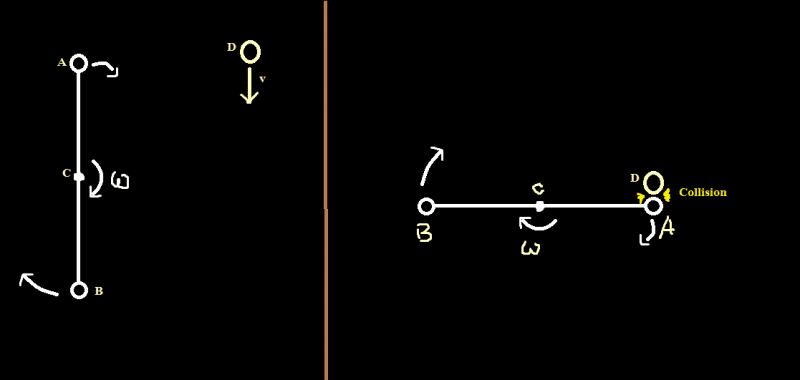# Applying law of momentum conservation

## Homework StatementSuppose, a rod of negligible mass has two balls(A & B) at its two ends. Both the balls have same mass m. The rod is rotating around its center C with a uniform angular velocity ##\omega##. Another ball D (mass M) has a velocity v. At some time, A and D collide with each other. Now, how to apply the law of momentum conservation in this case? (The actual problem was different. But I need to understand how to apply the law of momentum conservation in such case.)[/B]

## The Attempt at a Solution

In this case, there are both linear and angular momentum. That confuses me.
Let the length of the rod be 2R.
And the final angular velocity of the rod is ##\omega _f## and final velocity of D is ##v_f##;
Now, I thought,
## m \omega R - m \omega R + Mv = m \omega _f R - m \omega _f R + Mv_f##
So, it becomes, ## v = v_f ##
But, it's not true.

TSny
Homework Helper
Gold Member
It is important to specify as part of the problem statement whether the rod is rotating about a fixed axis at C or whether it initially rotating freely in space (not attached to a fixed axis of rotation).

Last edited:
Another thing to ask: do D and A have an elastic or inelastic collision?

Also, looking at:
$mωR − mωR +M = mω_fR − mω_fR +Mv_f$
I believe mωR should be added to the other mωR because they spin in the same direction, i.e. they have the same value for ω

It is important to specify as part of the problem statement whether the rod is rotating about a fixed axis at C or whether it initially rotating freely in space (not attached to a fixed axis of rotation).
Fixed axis.
Another thing to ask: do D and A have an elastic or inelastic collision?
Elastic collision.

TSny
Homework Helper
Gold Member
If the axis is fixed, then the total linear momentum of the system consisting of the rod and ball D will not be conserved. Can you see why?

If the axis is fixed, then the total linear momentum of the system consisting of the rod and ball D will not be conserved. Can you see why?
That's a very good point. There will be some force acting on the center C from outside. Then how to calculate the final angular velocity ?

TSny
Homework Helper
Gold Member
Besides linear momentum, what other type of momentum should you think about here?

Besides linear momentum, what other type of momentum should you think about here?
Surely, angular momentum. But, what is the angular momentum of D ?

TSny
Homework Helper
Gold Member
Did you cover the definition of angular momentum of a point particle?

Did you cover the definition of angular momentum of a point particle?
So, I think the equation will be,
## 2m \omega r^2 + Mvr = 2m \omega _f r^2 + Mv_f r##
And the equation for energy conservation,
##2\cdot \frac {1}{2} m r^2 \omega ^2+ \frac{1}{2} Mv^2 = 2\cdot \frac {1}{2} m r^2\omega _f ^2 + \frac{1}{2} Mv_f ^2 ##

TSny
Homework Helper
Gold Member
Looks good. (r = R)

Looks good. (r = R)
In the actual problem, the picture was in the vertical plane. The ball D was falling down with the accelaration of ##9.8 ms^{-2}##.
So, in this case, I think, there will be a torque because of collision and another torque which is equal to ##MgR##.
Is it so?

TSny
Homework Helper
Gold Member
Generally for collision problems you assume that the collision forces are much stronger than the forces of gravity. So you assume that you can neglect the gravity force during the collision. This is sometimes referred to as the "impulse approximation". If you did want to include the effect of the weight of D during the collision, then you would need to know the time duration of the collision in order to determine the angular impulse caused by Mg.

•arpon
Generally for collision problems you assume that the collision forces are much stronger than the forces of gravity. So you assume that you can neglect the gravity force during the collision. This is sometimes referred to as the "impulse approximation". If you did want to include the effect of the weight of D during the collision, then you would need to know the time duration of the collision in order to determine the angular impulse caused by Mg.
That's good!
Now, what would happen if the center was not fixed?

TSny
Homework Helper
Gold Member
Think about how many "unknowns" appear in the final state now that the entire rod can "recoil". How many conserved quantities are there?

I need to quit for the night. Will check back tomorrow.

Think about how many "unknowns" appear in the final state now that the entire rod can "recoil". How many conserved quantities are there?

I need to quit for the night. Will check back tomorrow.
I think, after collision, the rod will have two type of motion: translational and rotational.
Let, the final translational velocity of the rod be ##v_1## and final velocity of ball D be ##v_2## ; and final angular velocity of rod be ##\omega_1## ;
So, applying the law of (linear) momentum conservation:
##0 + Mv = 2mv_1 + Mv_2## (assuming the rod as a point particle at its center of mass)
And angular momentum conservation:
##2m\omega r^2 + Mvr = 2m \omega _1 r^2 + Mv_2r##
Energy conservation:
##\frac{1}{2} \cdot 2mr^2 \omega ^2 + \frac {1}{2} Mv^2 = \frac{1}{2} \cdot 2mr^2 \omega _1^2 + \frac {1}{2} \cdot 2mv_1^2+ \frac {1}{2} Mv_2^2##
Three unknowns : ##\omega _1, v_1, v_2## ; three equations ;
Looks good?

TSny
Homework Helper
Gold Member
Nice. Your equations look correct to me.